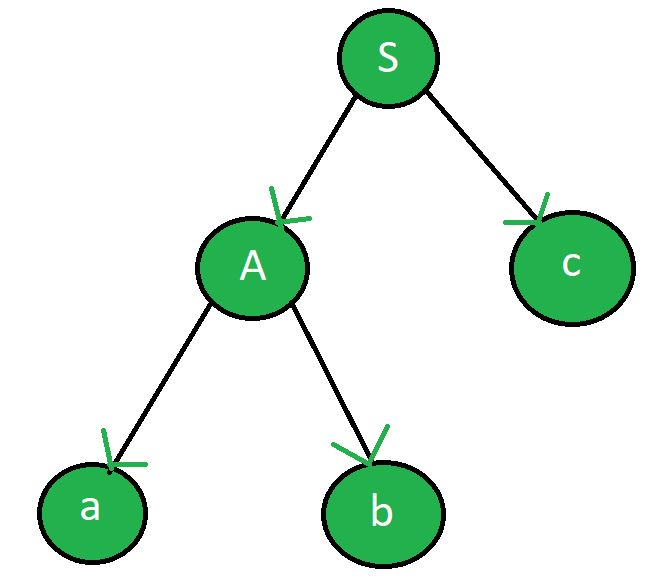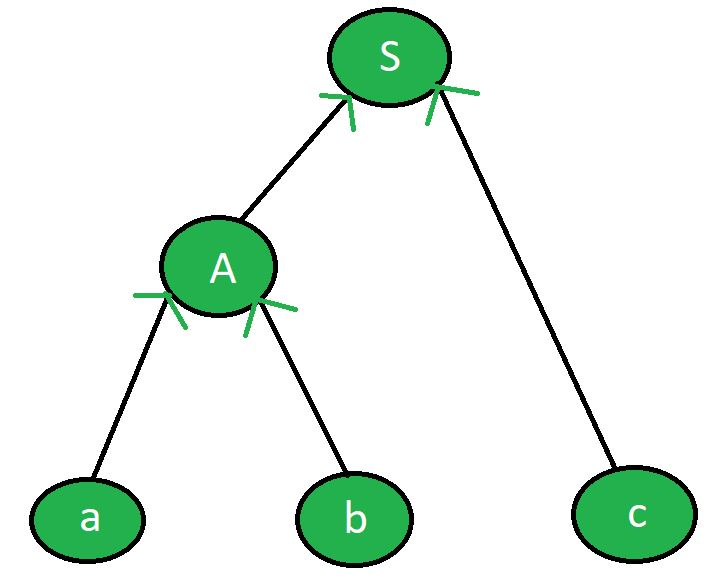Open In App

# Difference between LL and LR parser

LL Parser includes both the recursive descent parser and non-recursive descent parser. Its one type uses backtracking while another one uses parsing table. Theses are top down parser.
Example: Given grammar is

```S -> Ac
A -> ab ```

where S is start symbol, A is non-terminal and a, b, c are terminals.

Input string: abc
Parse tree generated by LL parser:LR Parser is one of the bottom up parser which uses parsing table (dynamic programming) to obtain the parse tree form given string using grammar productions.

Example: In the above example, parse tree generated by LR parser:Difference between LL and LR parser:

LL ParserLR Parser
First L of LL is for left to right and second L is for leftmost derivation.L of LR is for left to right and R is for rightmost derivation.
It follows the left most derivation.It follows reverse of right most derivation.
Using LL parser parser tree is constructed in top down manner.Parser tree is constructed in bottom up manner.
In LL parser, non-terminals are expanded.In LR parser, terminals are compressed.
Starts with the start symbol(S).Ends with start symbol(S).
Ends when stack used becomes empty.Starts with an empty stack.
Pre-order traversal of the parse tree.Post-order traversal of the parser tree.
Terminal is read after popping out of stack.Terminal is read before pushing into the stack.
It may use backtracking or dynamic programming.It uses dynamic programming.
LL is easier to write.LR is difficult to write.
Example: LL(0), LL(1)Example: LR(0), SLR(1), LALR(1), CLR(1)